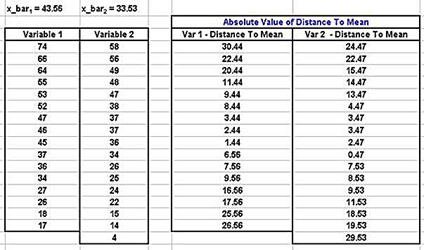## Thursday, May 29, 2014

### Levene’s + Brown-Forsythe Tests: F-Test Alternatives in Excel

This is one of the following three articles on F Tests in Excel in Excel

F-Test in 6 Steps in Excel 2010 and Excel 2013

Normality Testing For F Test In Excel 2010 and Excel 2013

Levene’s and Brown- Forsythe Tests: F-Test Alternatives in Excel

# Levene’s Test and the Brown-Forsythe Test in Excel – Nonparametric Alternatives to the F Test

The F Test is extremely sensitive to non-normality of either population. When normality of population of sample data cannot be confirmed, sample variances can be compared using the nonparametric Levene’s Test and also the nonparametric Brown-Forsythe Test.

It is often a good idea to perform at least one of these tests along with the F Test even when sample or population normality has been confirmed.

Levene’s Test and the Brown-Forsythe Test will be performed on the sample data as follows:

## Levene’s Test For Sample Variance Comparison in Excel

Levene’s Test is a hypothesis test commonly used to test for the equality of variances of two or more sample groups. Levene’s Test is more robust against non-normality of data than the F Test.

The Null Hypothesis of Levene’s Test is average distance to the sample mean is the same for each sample group. Acceptance of this Null Hypothesis implies that the variances of the sampled groups are the same. The distance to the mean for each data point of both samples is shown as follows:(Click On Image To See a Larger Version)

Levene’s Test involves performing Single-Factor ANOVA on the groups of distances to the mean. This can be easily implemented in Excel by applying the Excel data analysis tool ANOVA: Single Factor. Applying this tool on the above data produces the following output:(Click On Image To See a Larger Version)

The Null Hypothesis of Levene’s Test states that the average distance to the mean for the two groups are the same. Acceptance of this Null Hypothesis would imply that the sample groups have the same variances. The p Value shown in the Excel ANOVA output equals 0.6472. This is much larger than the Alpha (0.05) that is typically used for an ANOVA Test so the Null Hypothesis cannot be rejected.

We therefore conclude as a result of Levene’s Test that the variances are the same or, at least, that we don’t have enough evidence to state with 95 percent certainty that the variances are different. Levene’s Test is sensitive to outliers because relies on the sample mean, which can be unduly affected by outliers. A very similar nonparametric test called the Brown-Forsythe Test relies on sample medians and is therefore much less affected by outliers as Levene’s Test is or by non-normality as the F Test is.

## Brown-Forsythe Test For Sample Variance Comparison in Excel

The Brown-Forsythe Test is a hypothesis test commonly used to test for the equality of variances of two or more sample groups. The Null Hypothesis of the Brown-Forsythe Test is average distance to the sample median is the same for each sample group. Acceptance of this Null Hypothesis implies that the variances of the sampled groups are the same. The distance to the median for each data point of both samples is shown as follows:(Click On Image To See a Larger Version)

The Brown-Forsythe Test involves performing Single-Factor ANOVA on the groups of distances to the median. This can be easily implemented in Excel by applying the Excel data analysis tool ANOVA: Single Factor. Applying this tool on the above data produces the following output:(Click On Image To See a Larger Version)

The Null Hypothesis of the Brown-Forsythe Test states that the average distance to the median for the two groups are the same. Acceptance of this Null Hypothesis would imply that the sample groups have the same variances. The p Value shown in the Excel ANOVA output equals 0.6627. This is much larger than the Alpha (0.05) that is typically used for an ANOVA Test so the Null Hypothesis cannot be rejected.

Excel Master Series Blog Directory

Statistical Topics and Articles In Each Topic

• Histograms in Excel
• Bar Chart in Excel
• Combinations & Permutations in Excel
• Normal Distribution in Excel
• t-Distribution in Excel
• Binomial Distribution in Excel
• z-Tests in Excel
• t-Tests in Excel
• Hypothesis Tests of Proportion in Excel
• Chi-Square Independence Tests in Excel
• Chi-Square Goodness-Of-Fit Tests in Excel
• F Tests in Excel
• Correlation in Excel
• Pearson Correlation in Excel
• Spearman Correlation in Excel
• Confidence Intervals in Excel
• Simple Linear Regression in Excel
• Multiple Linear Regression in Excel
• Logistic Regression in Excel
• Single-Factor ANOVA in Excel
• Two-Factor ANOVA With Replication in Excel
• Two-Factor ANOVA Without Replication in Excel
• Randomized Block Design ANOVA in Excel
• Repeated-Measures ANOVA in Excel
• ANCOVA in Excel
• Normality Testing in Excel
• Nonparametric Testing in Excel
• Post Hoc Testing in Excel
• Creating Interactive Graphs of Statistical Distributions in Excel
• Solving Problems With Other Distributions in Excel
• Optimization With Excel Solver
• Chi-Square Population Variance Test in Excel
• Analyzing Data With Pivot Tables
• SEO Functions in Excel
• Time Series Analysis in Excel
• VLOOKUP